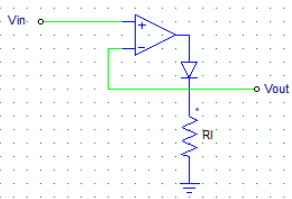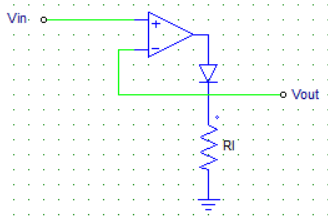Test: Types of Power Diodes

# Test: Types of Power Diodes

Test Description

## 20 Questions MCQ Test Power Electronics | Test: Types of Power Diodes

Test: Types of Power Diodes for Electrical Engineering (EE) 2023 is part of Power Electronics preparation. The Test: Types of Power Diodes questions and answers have been prepared according to the Electrical Engineering (EE) exam syllabus.The Test: Types of Power Diodes MCQs are made for Electrical Engineering (EE) 2023 Exam. Find important definitions, questions, notes, meanings, examples, exercises, MCQs and online tests for Test: Types of Power Diodes below.
Solutions of Test: Types of Power Diodes questions in English are available as part of our Power Electronics for Electrical Engineering (EE) & Test: Types of Power Diodes solutions in Hindi for Power Electronics course. Download more important topics, notes, lectures and mock test series for Electrical Engineering (EE) Exam by signing up for free. Attempt Test: Types of Power Diodes | 20 questions in 20 minutes | Mock test for Electrical Engineering (EE) preparation | Free important questions MCQ to study Power Electronics for Electrical Engineering (EE) Exam | Download free PDF with solutions
 1 Crore+ students have signed up on EduRev. Have you?
Test: Types of Power Diodes - Question 1

### Shown below is the diagram of an ideal super diode. When the input voltage Vin is negative, then the output voltage Vout = ?​Detailed Solution for Test: Types of Power Diodes - Question 1

When the input voltage is negative, there would be a negative voltage on the diode so it works like an open circuit. Hence no current flows through the load and Vout is zero.

Test: Types of Power Diodes - Question 2

### In order to reduce the reverse recovery time of the diodes, __________ is carried out.

Detailed Solution for Test: Types of Power Diodes - Question 2

Platinum & gold doping improves the performance of the devices.

Test: Types of Power Diodes - Question 3

### Which of the following diodes uses a metal-semiconductor junction?

Detailed Solution for Test: Types of Power Diodes - Question 3

Schottky diode uses a Al-Semiconductor junction.

Test: Types of Power Diodes - Question 4

Which of the below mentioned statements is false regarding Schottky diodes?

Detailed Solution for Test: Types of Power Diodes - Question 4

The majority charge carriers in a Schottky diode are electrons not holes.

Test: Types of Power Diodes - Question 5

A Schottky diode _____

Detailed Solution for Test: Types of Power Diodes - Question 5

Due to the metal-silicon junction there are no stored charges hence, no reverse recovery time, due to which the switching is faster.

Test: Types of Power Diodes - Question 6

Which of the following are/is the majority charge carriers in a Schottky diode?

Detailed Solution for Test: Types of Power Diodes - Question 6

The metal has no holes hence major(almost full) current flows due to the electrons only.

Test: Types of Power Diodes - Question 7

In a Schottky diode, the silcon is usually

Detailed Solution for Test: Types of Power Diodes - Question 7

Usually only n-type silicon is used because the p-type has certain limitations.

Test: Types of Power Diodes - Question 8

As compared to a p-n junction diode(of the same rating), a Schottky diode has ___________

Detailed Solution for Test: Types of Power Diodes - Question 8

Due to the metal-silicon junction there are no stored charges, hence no reverse recovery time due to which the switching is faster.

Test: Types of Power Diodes - Question 9

A Schottky diode has __________

Detailed Solution for Test: Types of Power Diodes - Question 9

Schottky diode uses a Al-Semiconductor junction.

Test: Types of Power Diodes - Question 10

A Schottky diode can be switchd off much faster than an equivalent p-n junction diode due to its

Detailed Solution for Test: Types of Power Diodes - Question 10

Due to the metal-silicon junction there are no stored charges, hence no reverse recovery time due to which the switching is faster.

Test: Types of Power Diodes - Question 11

Ideally the voltage drop across a conducting diode must be

Detailed Solution for Test: Types of Power Diodes - Question 11

Ideal conduction = No losses.

Test: Types of Power Diodes - Question 12

When reverse breakdown occurs in a diode

Detailed Solution for Test: Types of Power Diodes - Question 12

Recall the I-V curve of a diode in the 3rd quadrant.

Test: Types of Power Diodes - Question 13

Schottky diodes are also called as

Detailed Solution for Test: Types of Power Diodes - Question 13

Due to the metal used to carry the current, it is also called as a hot carrier diode.

Test: Types of Power Diodes - Question 14

In a Schottky diode, the aluminum metal acts as a __________

Detailed Solution for Test: Types of Power Diodes - Question 14

The Al (Metal) always acts as the anode.

Test: Types of Power Diodes - Question 15

If the doping levels of the semiconductor is increased, then the width of the depletion layer

Detailed Solution for Test: Types of Power Diodes - Question 15

Higher the doping, more the number of charge carrier available to neutralize the opposite charges on the junction.

Test: Types of Power Diodes - Question 16

As compared to a p-n junction device of equal rating, the Schottky diode has

Detailed Solution for Test: Types of Power Diodes - Question 16

Low reverse voltage rating is the only drawback against a p-n junction diode.

Test: Types of Power Diodes - Question 17

In a Schottky diode, the silicon layer acts as a _____________

Detailed Solution for Test: Types of Power Diodes - Question 17

The metal acts as the anode and the semiconductor as a cathode.

Test: Types of Power Diodes - Question 18

In a certain power electronics application, it is required that the voltage at the load terminals is to be kept within a certain range of voltages only. Among the device listed below, which would be the most ideal choice for this application?

Detailed Solution for Test: Types of Power Diodes - Question 18

Zener diode is used as a voltage regulating device.

Test: Types of Power Diodes - Question 19

Shown below is the diagram of an ideal super diode. When the input voltage Vin is positive, then the output voltage Vout = ?​Detailed Solution for Test: Types of Power Diodes - Question 19

Input is positive, hence it is amplified by the operational amplifier which switches the diode on. Current flows through the load & because of the feedback, the output voltage is equal to the input voltage.

Test: Types of Power Diodes - Question 20

Zener diodes allow a current to flow in the reverse direction, when the

Detailed Solution for Test: Types of Power Diodes - Question 20

Zener diode has voltage regulating property. When voltage reaches above a certain value (Zener voltage), current starts to flow in the reverse direction.

## Power Electronics

5 videos|39 docs|63 tests
 Use Code STAYHOME200 and get INR 200 additional OFF Use Coupon Code
Information about Test: Types of Power Diodes Page
In this test you can find the Exam questions for Test: Types of Power Diodes solved & explained in the simplest way possible. Besides giving Questions and answers for Test: Types of Power Diodes, EduRev gives you an ample number of Online tests for practice

## Power Electronics

5 videos|39 docs|63 tests